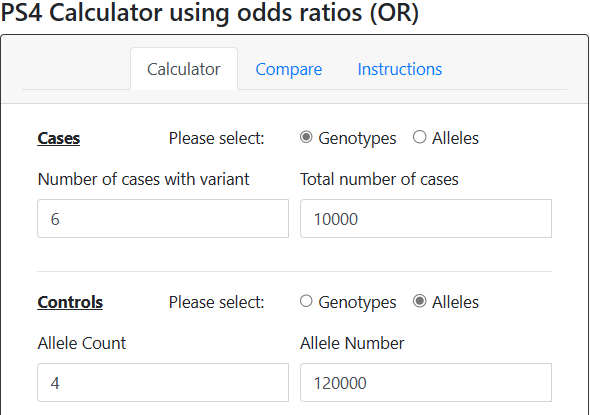#### PS4 Calculator using odds ratios (OR)

Cases

Controls
OR ≥
%CI
OR ≥
%CI
OR ≥
%CI

Created by: M. Bowman, Oxford Regional Genetics Laboratory, UK.

Email: m.bowman@ouh.nhs.uk

This calculator can be used to calculate an odds ratio (OR) which can inform the application of the ACMG PS4 criterion for variants in genes related to autosomal dominant disorders. This calculator is not intended to be used in the context of autosomal recessive disorders.

The OR is the ratio of the odds of a disease / phenotype in exposed versus non-exposed individuals. In genetic terms, it is an approximation for the relative risk (RR) of developing a disease / phenotype associated with having a particular genotype or allele as compared with not having that genotype or allele. An OR of >1 indicates an increased risk; an OR of <1 indicates decreased risk.

###### Inputting case and control data
The calculator accepts either genotypes or alleles as input.

When inputting genotypes users should add the total number of cases or controls with the variant (heterozygous or homozygous) and the total number of cases or controls tested.

When inputting alleles users should add the total variant allele count (AC) and total allele number (AN) for cases or controls. Please note, this option does not make a distinction between heterozygous or homozygous counts and therefore assumes occurrences are heterozygous.

###### Example
6 out of 10,000 individuals in a case cohort are heterozygous for a variant.

The allele count (AC) for that variant in gnomAD is 4 and the allele number (AN) is 120,000.###### Calculating the Odds ratio with confidence intervals
The calculator converts the input data to genotype counts to generate the following values in a 2×2 table (which can viewed by clicking ‘Show 2×2 table’):

a = Number of cases with the variant
b = Number of cases without the variant
c = Number of controls with the variant
d = Number of controls without the variant

The OR is calculated as follows:
OR = (a / b) / (c / d)

An OR should always be interpreted in the context of confidence intervals (CIs). The calculation of an odds ratio is based on a sample of cases and a sample of controls, and confidence intervals provide an indication of the likely range of the ‘true’ value of an OR in an entire population (rather than just the sample). Confidence intervals are calculated according to the following formula:

Upper CI = e [log(OR) + z * √(1/a + 1/b + 1/c + 1/d)]
Lower CI = e [log(OR) - z * √(1/a + 1/b + 1/c + 1/d)]

In this formula, the value of z determines the level of confidence calculated. For 95% confidence intervals, z = 1.96. For 90% confidence, z = 1.645, and for 99% confidence, z = 2.576.

An OR should only be considered to represent a significant increase in risk if the lower confidence interval is greater than 1.

###### Selecting levels for PS4 criterion
The application of PS4 at various strength levels should be determined by the calculated value of the lower confidence interval. Users can select OR thresholds for each criterion level ‘Strong’, ‘Moderate’ or ‘Supporting’, and the % confidence interval (CI) they wish to use.

###### Example
In the ClinGen guidelines for variants detected in sarcomeric genes in the context of inherited cardiomyopathy, it has been proposed that PS4 can be applied at supporting, moderate, or strong level if the 95% lower confidence interval is ≥5, ≥10, or ≥20 respectively.

6 in 6,000 case genotypes vs 2 in 60,000 control genotypes gives an odds ratio of 30.03 (95% CI = 6.06-148.81).

The lower bound 95% CI is greater than 5 (95% CI = 6.06). Therefore, PS4 is set to PS4_Supporting.

Created by: M. Bowman, Oxford Regional Genetics Laboratory, UK.

Email: m.bowman@ouh.nhs.uk